2021 NFL MVP Odds
+175
1.75 to 1Tom Brady
30.6% implied probability

+600
6 to 1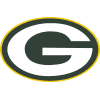Aaron Rodgers
12.0% implied probability

+750
7.5 to 1Kyler Murray
9.9% implied probability

+1000
10 to 1Josh Allen
7.6% implied probability

+1200
12 to 1Dak Prescott
6.5% implied probability

+1400
14 to 1Patrick Mahomes
5.6% implied probability

+1600
16 to 1Matthew Stafford
4.9% implied probability

+1600
16 to 1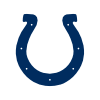Jonathan Taylor
4.9% implied probability

+1800
18 to 1Justin Herbert
4.4% implied probability

+2500
25 to 1Lamar Jackson
3.2% implied probability

+4000
40 to 1Cooper Kupp
2.1% implied probability

+6500
65 to 1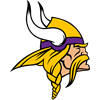Kirk Cousins
1.3% implied probability

+8000
80 to 1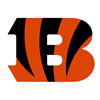Joe Burrow
1.0% implied probability

+10000
100 to 1Jalen Hurts
0.8% implied probability

+10000
100 to 1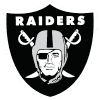Derek Carr
0.8% implied probability

+10000
100 to 1Mac Jones
0.8% implied probability

+10000
100 to 1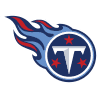Ryan Tannehill
0.8% implied probability

+10000
100 to 1James Conner
0.8% implied probability

+20000
200 to 1Carson Wentz
0.4% implied probability

+20000
200 to 1Teddy Bridgewater
0.4% implied probability

+25000
250 to 1Austin Ekeler
0.3% implied probability

+25000
250 to 1Justin Jefferson
0.3% implied probability

+50000
500 to 1Davante Adams
0.2% implied probability